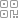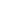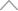5 8 8 6 2 5 8

# Pandas数据排序，人人都能学会的几种方法

Pandas 可以说是 在Python数据科学领域应用最为广泛的工具之一。

Pandas是一种高效的数据处理库，它以

dataframe

series

sort_index

和

sort_values

## 01 按索引排序

### 数据准备

pandas

numpy

，首先导入 Python 库，如下：

import pandas as pd

import numpy as np

print(f'pandas version: {pd.__version__}'

# pandas version 1.3.2

data = {

'brand':['Python数据之道','价值前瞻','菜鸟数据之道','Python','Java'],

'B':[4,6,8,12,10],

'A':[10,2,5,20,16],

'D':[6,18,14,6,12],

'years':[4,1,1,30,30],

'C':[8,12,18,8,2],

}

index = [9,3,4,5,2]

df = pd.DataFrame(data=data,index=index)

df### 按行索引排序

sort_index()

sort_indexascending

ascending=True

，为升序排列。

ascending=False### 按列的名称排序

axis=1ascending• Pandas实用技能，将列（column）排序的几种方法

## 02 按数值排序

sort_values()

### 按单个列的值排序

sort_values()

ascending### 按多个列的值排序

sort_values()ascending

years

B### 选择排序算法numpy 文档地址：

https://numpy.org/doc/stable/reference/generated/numpy.sort.html#numpy.sort### 忽略索引

ignore_index### inplace

inplace

inplace = True

：不创建新的对象，直接对原始对象进行修改；默认是

False

，即创建新的对象进行修改，原对象不变，和深复制和浅复制有些类似。### 缺失值

data = {

'brand':['Python数据之道','价值前瞻','菜鸟数据之道','Python','Java'],

'B':[4,6,8,np.nan,12],

'A':['Lemon','emma','ZW','app','John'],

'D':[6,18,14,6,12],

'years':[4,1,1,30,30],

'C':[8,12,18,8,2],

}

index = [9,3,4,5,2]

df1 = pd.DataFrame(data=data,index=index)

df1### key 参数## 新知精选

IT研发知识▪优秀创作者

### 优选课程 新知学院

•扫码下载商业新知APP~

•回到顶部